STICK FLICK PENALTY KICK GAME 2: LET'S SHOOT THE FOOTBALL

In the previous post we have set up the football ground and we are all set to shoot and save the penalties. In this part of this tutorial series, we will add shooting capabilities to both the player and the opponent.

Note that you need Unity Remote to test this post.

To get started with this, we need to create a new C# script named SwipeControl. But before that just for the sake of neatness, we will create a New Folder and name it as Scripts under the Assets folder. Place the SwipeControl script in this folder. Attach the C# script to the Football gameobject. Double click on the script to open it so that we can start adding some logic to it.

First of all we will establish some variables

```//variables for swipe input detection
private Vector3 fp; //First finger position
private Vector3 lp; //Last finger position
private float dragDistance;  //Distance needed for a swipe to register

//variables for determining the shot power and position
public float power;  //power at which the ball is shot
private Vector3 footballPos; //initial football position for replacing the ball at the same posiiton
private float factor = 34f; // keep this factor constant, also used to determine force of shot

public bool canShoot = true;  //flag to check if shot can be taken
public int scorePlayer = 0;  //score of player
public int scoreOpponent = 0; //score of oponent
public int turn = 0;   //0 for striker, 1 for goalie
public bool isGameOver = false; //flag for game over detection
Vector3 oppKickDir;   //direction at which the ball is kicked by opponent
public int shotsTaken = 0;  //number of rounds of penalties taken
private bool returned = true;  //flag to check if the ball is returned to its initial position
public bool isKickedPlayer = false; //flag to check if the player has kicked the ball
public bool isKickedOpponent = false; //flag to check if the opponent has kicked the ball
```
Now those are one heck of a variables. Aren't they? But hey! We need them!

The Start function will look like

```void Start(){
Time.timeScale = 1;    //set it to 1 on start so as to overcome the effects of restarting the game by script
dragDistance = Screen.height*20/100; //20% of the screen should be swiped to shoot
Physics.gravity = new Vector3(0, -20, 0); //reset the gravity of the ball to 20
footballPos = transform.position;  //store the initial position of the football
}
```
- The Time.timeScale is set to 1 on start as will set it to 0 once the game is over so as to stop the world.
- The player needs to swipe for a minimum distance of 20% of the phone screen if the is to shoot the football.
- We have increased the gravity so as to make sure that the ball behaves in a normal manner
- The initial position of the football is stored to reposition it after every shot is taken.

The Update function is where most of the logic will be involved so we will create functions which will be called from it so as to make it more readable.

```// Update is called once per frame
void Update()
{
if(returned){     //check if the football is in its initial position
if(turn==0 && !isGameOver){ //if its users turn to shoot and if the game is not over
playerLogic();   //call the playerLogic fucntion
}
else if(turn==1 && !isGameOver){ //if its opponent's turn to shoot
opponentLogic(); //call the respective function
}
}
}
```

Now that was pretty simple, eh! But then, the whole logic is pending. Phew! Am I not tired of typing?

```void playerLogic(){
//Examine the touch inputs
foreach (Touch touch in Input.touches)
{
if (touch.phase == TouchPhase.Began)
{
fp = touch.position;
lp = touch.position;
}

if (touch.phase == TouchPhase.Ended)
{
lp = touch.position;
//First check if it's actually a drag

if (Mathf.Abs(lp.x - fp.x) > dragDistance || Mathf.Abs(lp.y - fp.y) > dragDistance)
{   //It's a drag
//x and y repesent force to be added in the x, y axes.
float x = (lp.x - fp.x) / Screen.height * factor;
float y = (lp.y-fp.y)/Screen.height*factor;
//Now check what direction the drag was
//First check which axis
if (Mathf.Abs(lp.x - fp.x) > Mathf.Abs(lp.y - fp.y))
{   //If the horizontal movement is greater than the vertical movement...

if ((lp.x>fp.x) && canShoot)  //If the movement was to the right)
{   //Right move
}
else
{   //Left move
}
}
else
{   //the vertical movement is greater than the horizontal movement
if (lp.y>fp.y)  //If the movement was up
{   //Up move
}
else
{   //Down move

}
}
}
canShoot = false;
returned = false;
isKickedPlayer = true;
StartCoroutine(ReturnBall());
}
else
{   //It's a tap

}
}
}
```

Now the above function looks very long and complex. But trust me, it is not.
As we know that if turn is 0, we are supposed to be the striker and if it is 1, the opponent is the striker and we, the goalie.
Hence, the above function is called whenever turn is 0.

We basically check for genuine swipe here in the above function and also the swipe direction. Based on the swipe direction, we add force to the football in that respective direction. The foreach loop can be removed if you do not want to detect more than one swipe.

We catch hold of the first touch position when the swipe has begun. Meanwhile, the last touch is caught hold of in the end phase of the touch.
(Note: We have assigned lp i.e., the last touch position in the touch began phase itself, just to make sure that even a tap is detected)

Further, we check for the genuine swipe in the line 15. If it is a swipe, we dig on further to check the direction of the drag, videlicet, horizontal or vertical.

Once the direction is determined, we check if the swipe is left or right (horizontal), up or down for Vertical.

The lines

```float x = (lp.x - fp.x) / Screen.height * factor;
float y = (lp.y – fp.y) / Screen.height*factor;
```

We have divided by the Screen.height so the force added will not be Screen size dependent. To make it clear, the force added and the direction in which the ball is shot is the same regardless of what screen sized phone you play this game in.

For the right swipe and left swipe I have added a constant y and z values as 10 and 15 as I found them to be good enough so as to make the shoot look real. (Try modifying them)

For the Vertical swipe and the diagonal swipes, we need to determine the x and y values from the swipe itself. I have assigned the z value as a constant value (15) as the swipe on the screen is a 2D and you won't get a z axis value from it.

The x and y values are calculated based on the swipe distance, more the swipe distance, more the force.

The below flags are pretty self explanatory, I believe

```canShoot = false;
returned = false;
isKickedPlayer = true;
```

Once these are set, we call a Coroutine to add some delay before the ball is returned to its initial position.

```StartCoroutine(ReturnBall());
```

The ReturnBall function is as follows:

```IEnumerator ReturnBall() {
yield return new WaitForSeconds(5.0f);  //set a delay of 5 seconds before the ball is returned
rigidbody.velocity = Vector3.zero;   //set the velocity of the ball to zero
rigidbody.angularVelocity = Vector3.zero;  //set its angular vel to zero
transform.position = footballPos;   //re positon it to initial position
//take turns in shooting
if(turn==1)
turn=0;
else if(turn==0)
turn=1;
canShoot =true;     //set the canshoot flag to true
returned = true;     //set football returned flag to true as well
}
```

Next we will write a function for the opponent kick as follows:

```void opponentLogic(){
//check for screen tap
int fingerCount = 0;
foreach (Touch touch in Input.touches) {
if (touch.phase != TouchPhase.Ended && touch.phase != TouchPhase.Canceled)
fingerCount++;
}
//if tapped, the opponent will shoot the football after some time delay as mentioned below
if(fingerCount>0){
isKickedOpponent = true;  //set opponent kicked to true
shotsTaken++;    //increase set of penalty taken
returned = false;
StartCoroutine(ReturnBall()); //return the ball back to its initial position
}
}
```

The above function detects a tap on the screen and shoots the ball based on the force added in the DelayAdd function. The delay is added so as to give some time to the player to guess the direction in which the ball is shot.

The DelayAdd fucntion is as below:

```IEnumerator DelayAdd() {
yield return new WaitForSeconds(0.2f);  //I have added a delay of 0.2 seconds
oppKickDir = new Vector3(Random.Range(-4f, 4f), Random.Range(5f, 10f), Random.Range(6f, 12f));     //generate a random x and y value in the range mentioned
}
```

Feel free to modify the delay and the random value ranges of x and y to your needs.
Note that the Force mode her is impulse, as we want it to be a short burst of force.

Set the value of Power in the Inspector to 34.

The complete SwipeControl script will look like

```using UnityEngine;
using System.Collections;
using System.Collections.Generic;

public class SwipeControl : MonoBehaviour
{
//variables for swipe input detection
private Vector3 fp; //First finger position
private Vector3 lp; //Last finger position
private float dragDistance;  //Distance needed for a swipe to register

//variables for determining the shot power and position
public float power;  //power at which the ball is shot
private Vector3 footballPos; //initial football position for replacing the ball at the same posiiton
private float factor = 34f; // keep this factor constant, also used to determine force of shot

public bool canShoot = true;  //flag to check if shot can be taken
public int scorePlayer = 0;  //score of player
public int scoreOpponent = 0; //score of oponent
public int turn = 0;   //0 for striker, 1 for goalie
public bool isGameOver = false; //flag for game over detection
Vector3 oppKickDir;   //direction at which the ball is kicked by opponent
public int shotsTaken = 0;  //number of rounds of penalties taken
private bool returned = true;  //flag to check if the ball is returned to its initial position
public bool isKickedPlayer = false; //flag to check if the player has kicked the ball
public bool isKickedOpponent = false; //flag to check if the opponent has kicked the ball

void Start(){
Time.timeScale = 1;    //set it to 1 on start so as to overcome the effects of restarting the game by script
dragDistance = Screen.height*20/100; //20% of the screen should be swiped to shoot
Physics.gravity = new Vector3(0, -20, 0); //reset the gravity of the ball to 20
footballPos = transform.position;  //store the initial position of the football
}

// Update is called once per frame
void Update()
{
if(returned){     //check if the football is in its initial position
if(turn==0 && !isGameOver){ //if its users turn to shoot and if the game is not over
playerLogic();   //call the playerLogic fucntion
}
else if(turn==1 && !isGameOver){ //if its opponent's turn to shoot
opponentLogic(); //call the respective function
}
}
}

void playerLogic(){
//Examine the touch inputs
foreach (Touch touch in Input.touches)
{
if (touch.phase == TouchPhase.Began)
{
fp = touch.position;
lp = touch.position;
}

if (touch.phase == TouchPhase.Ended)
{
lp = touch.position;
//First check if it's actually a drag

if (Mathf.Abs(lp.x - fp.x) > dragDistance || Mathf.Abs(lp.y - fp.y) > dragDistance)
{   //It's a drag

//x and y repesent force to be added in the x, y axes.
float x = (lp.x - fp.x) / Screen.height * factor;
float y = (lp.y-fp.y)/Screen.height*factor;
//Now check what direction the drag was
//First check which axis
if (Mathf.Abs(lp.x - fp.x) > Mathf.Abs(lp.y - fp.y))
{   //If the horizontal movement is greater than the vertical movement...

if ((lp.x>fp.x) && canShoot)  //If the movement was to the right)
{   //Right move
}
else
{   //Left move
}
}
else
{   //the vertical movement is greater than the horizontal movement
if (lp.y>fp.y)  //If the movement was up
{   //Up move
}
else
{   //Down move

}
}
}
canShoot = false;
returned = false;
isKickedPlayer = true;
StartCoroutine(ReturnBall());
}
else
{   //It's a tap

}
}
}

IEnumerator ReturnBall() {
yield return new WaitForSeconds(5.0f);  //set a delay of 5 seconds before the ball is returned
rigidbody.velocity = Vector3.zero;   //set the velocity of the ball to zero
rigidbody.angularVelocity = Vector3.zero;  //set its angular vel to zero
transform.position = footballPos;   //re positon it to initial position
//take turns in shooting
if(turn==1)
turn=0;
else if(turn==0)
turn=1;
canShoot =true;     //set the canshoot flag to true
returned = true;     //set football returned flag to true as well
}

void opponentLogic(){
//check for screen tap
int fingerCount = 0;
foreach (Touch touch in Input.touches) {
if (touch.phase != TouchPhase.Ended && touch.phase != TouchPhase.Canceled)
fingerCount++;
}
//if tapped, the opponent will shoot the football after some time delay as mentioned below
if(fingerCount>0){
isKickedOpponent = true;  //set opponent kicked to true
shotsTaken++;    //increase set of penalty taken
returned = false;
StartCoroutine(ReturnBall()); //return the ball back to its initial position
}
}

yield return new WaitForSeconds(0.2f);  //I have added a delay of 0.2 seconds
oppKickDir = new Vector3(Random.Range(-4f, 4f), Random.Range(5f, 10f), Random.Range(6f, 12f));     //generate a random x and y value in the range mentioned
}

}
```

See you around

Stick Flick Penalty Kick Game 1: The Football Ground
Stick Flick Penalty Kick Game 3: Add GoalLine Technology
Stick Flick Penalty Kick Game 4: Goalkeeper Movement
Stick Flick Penalty Kick Game 5: Who Is The Winner?

Sujit Horakeri is a game freak just like any other next door guy you would come across. He is a Web Developer by Profession, Game Developer by Choice.
Blogger

1.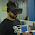hey, can u tell how football can make curve if swiped harder by player i hope u get point

1.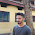Well, there are many ways to do it. Its not simple though. Either you can use Bezier curves to add a curl to the ball or you can simple apply a impulse force along the x axis in FixedUpdate so that the ball curls... Both can be equally effective, as I said, it's how you detect the curved swipe and add the curl to the ball.

2.I followed your steps one by one, and I get this error everytime I try to flick the ball:

MissingComponentException: There is no 'Rigidbody' attached to the "Football" game object, but a script is trying to access it.
You probably need to add a Rigidbody to the game object "Football". Or your script needs to check if the component is attached before using it.
SwipeControl.playerLogic () (at Assets/Scripts/SwipeControl.cs:90)
SwipeControl.Update () (at Assets/Scripts/SwipeControl.cs:43)

1.Make sure the football has a Rigidbody component attached to it. If not attach one by clicking on Add Component -> Physics -> Rigidbody

2.Hey, Thanks a lot for the help, it works now!

3.HI very nice tutorial. What if i only want to addforce once? and not constantly so that after some time object stops.

1.You can use the ForceMode.Impulse to add a force once... Check out the unity doc for more info..

4.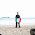So far so good, im making a project like this, but working in unity 5.
Everything works, only some changes in the API of the way to access to the components of a game object.

For example
rigidbody.velocity = Vector3.zero;
now the correct form to do it is:
GetComponent().velocity = Vector3.zero;

Great tutorial :3

5.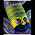i want to add force on curved swipe.
i mean, if i give a curved swipe as input, ball should travel a on a swinged path according to curve of swipe instead of goung in a straight direction.
what to add next in it?

using UnityEngine;
using System.Collections;
using System.Collections.Generic;

public class SwipeControl : MonoBehaviour {

public GameObject ball;
Vector3 firstpos, lastpos;
Vector3 force;
Vector3 startpos;
Vector3 swingforce;

float starttime, endtime;
float dragdistance;

public bool returned = true;
public bool drag = false;

void Start()
{
dragdistance = Screen.height * 10 / 100;
startpos = ball.transform.position;
}

void Update()
{
if (returned)
{
Player ();
}
}

void Player()
{

if (Input.GetMouseButtonDown (0))
{
starttime = Time.time;
firstpos = Input.mousePosition;
}

if (Input.GetMouseButton(0))
{

}

if (Input.GetMouseButtonUp (0))
{
drag = false;

endtime = Time.time;
lastpos = Input.mousePosition;

Vector3 distance = lastpos - firstpos;
distance.z = distance.magnitude;

if (distance.x > dragdistance || distance.y > dragdistance)
{

Vector3 force = (distance / ((endtime - starttime)/0.5f));

if (force.x > 400.0f)
{
force.x = 400.0f;
}
else if(force.x < -200.0f)
{
force.x = -200.0f;
}

if (force.y > 400.0f)
{
force.y = 400.0f;
}
else if(force.y < 200.0f)
{
force.y = 200.0f;
}

if (force.z > 400.0f)
{
force.z = 400.0f;
}
else if(force.z < 320.0f)
{
force.z = 320.0f;
}

returned = false;
StartCoroutine (Returnball ());
}
}
}

IEnumerator Returnball()
{
yield return new WaitForSeconds (3.0f);
ball.rigidbody.velocity = Vector3.zero;
ball.rigidbody.angularVelocity = Vector3.zero;
ball.transform.position = startpos;
returned = true;
}
}

1.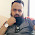please post code for goolkeeper too

6.Thank you very much for this post !! :D
Very tutorial, i´m having some problems because i´m at Unity 5.3 and i have to optimize the scripts...

7.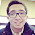Hello I hope you can answer me. I have a question, its regarding this post. When I play the scene the ball never moves. I don't know why. Could you help me? Thanks and good post btw.

1.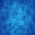This comment has been removed by the author.

2.hi there did u figure out the problem? i am having the same problem.

8.I am unable to shoot the ball in the editor, but I can in the mobile. Why is that ?
Also the ball moves very slowly. How can I speed it up ?
Thanks.

9.hi there when i play the scene the ball does not move.can you help me please? love this tutorial 10/10

10.ball cannot swipe in game

11.ball cannot swipe in game

12.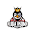its doesnt work on unity 2018?

13.6 error are found

14.15.16.17.